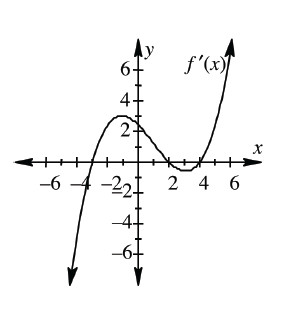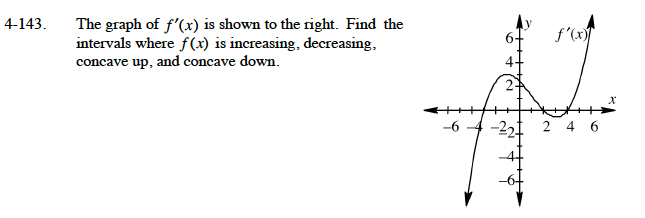### Home > CALC > Chapter 4 > Lesson 4.4.2 > Problem4-143

4-143.
1. The graph of f ′(x) is shown below. Find the intervals where f(x) is increasing, decreasing, concave up, and concave down. Homework Help ✎We are looking at a graph of f '(x), but being asked about the graph of f(x).